### First Order Reaction in Two Mixed Flow Reactors in Series

A first order reaction is to be treated in a series of two mixed reactors. Show that the total volume of the two reactors is minimum when the reactors are equal in size.

Assumptions:

1. Constant density system
2. Isothermal operation

For the CSTR in series,

Vi = FAo(XA,i - XA,i-1)/(-rA)i → 1

For two reactors,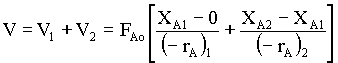→ 2

From the rate equation,

(-rA)1 = k CAo (1 - XA1) → 3

(-rA)2 = k CAo (1 - XA2) → 4

substituting 3 and 4 in equn.2,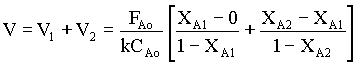→ 5

for minimum V,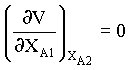→ 6

from equn.5 and 6, we obtain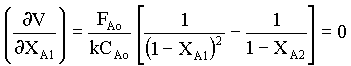from which,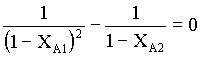1/( 1- XA1)2 = 1/(1 - XA2)

( 1- XA1)2 = (1 - XA2)

XA2 = 1 - ( 1- XA1)2 = 1 -1 + 2XA1 - XA12 = 2XA1 - XA12 = XA1(2 - XA1) → 7

Substituting for XA2 from equn.7 in equn.5 for the last term (V2),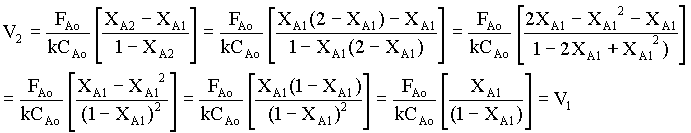That is, for a first order equation, the two stages must be of equal size to minimize V. The proof can be extended to an N-stage CSTR. For other orders of reaction, this result is approximately correct. The conclusion is that tanks in series should all be the same size, which accords with ease of fabrication.